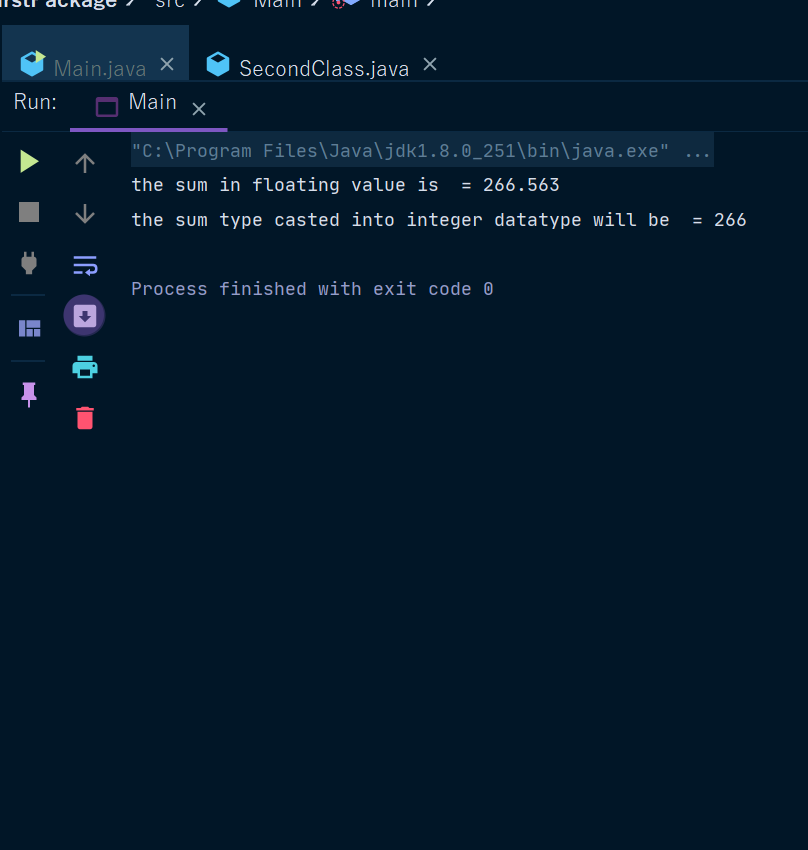Java float Type

# Java float Type

Float Type In Java

In this tutorial, you will learn about float type in Java with hepl of examples:- float is a keyword used to declare float data type variables by putting float keyword before the identifier or the variable name.

Floating-point numbers, also known as real numbers, are used when evaluating expressions that require fractional precision.

For example, calculations such as square root, or transcendentals such as sine and cosine, result in a value whose precision requires a floating-point type.
Java implements the standard (IEEE–754) set of floating-point types and operators. There are two kinds of floating-point types, float and double, which represent single- and double-precision numbers, respectively

You should use a floating-point type whenever you need a number with a decimal, such as 3.14 or 32.1456.

We use float data type when the space required is 4 bytes or less, it the space requirement is more we use double, and put f at the end of the value.

The type float specifies a single-precision value that uses 32 bits of storage.
Single precision is faster on some processors and takes half as much space as double-precision, but will become imprecise when the values are either very large or very small.
Variables of type float are useful when you need a fractional component, but don’t require a large degree of precision.

For example, the float can be useful when representing dollars and cents.
Here are some example float variable declarations -

Example 1 -

 float num =3.14f; System.out.println(num);

Example 2 -

 import second.*; class Main{   public static void main(String[] args) {       float a = 232.22f;   float b = 34.343f; float sum = (a+b); System.out.println("the sum in floating value is  = "+sum); System.out.println("the sum type casted into integer datatype will be  = "+(int)sum);   } }

Output  -